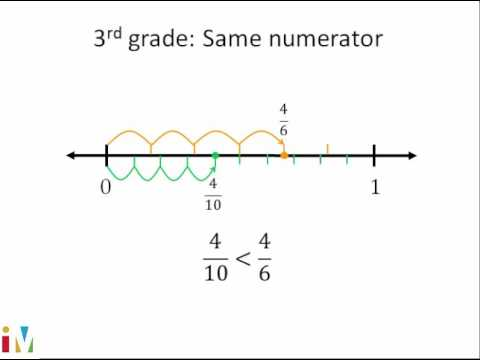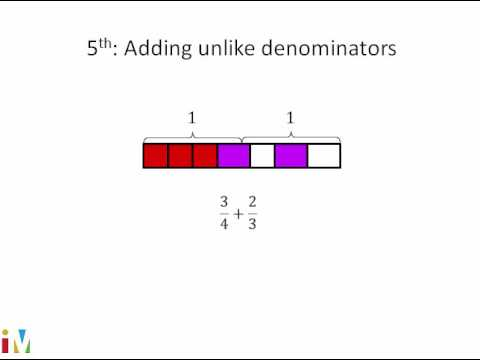Video

# Multiplying 2 fractions: 5/6 × 2/3 (Full video)

Description: Let's practice multiplying two fractions together. We'll learn to simplify the answer, too. We're asked to multiply 5/6 times 2/3 and then simplify our answer. You could've said, well, I have a 2 in the numerator and I have something divisible by 2 into the denominator, so let me divide the numerator by 2, and this becomes a 1. You could actually do it right here.

### Other videos you might be interested in### Comparing Fractions

#### Illustrative Mathematics